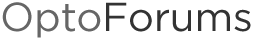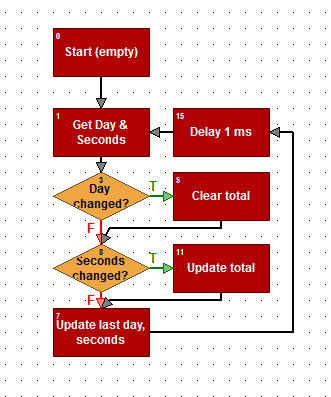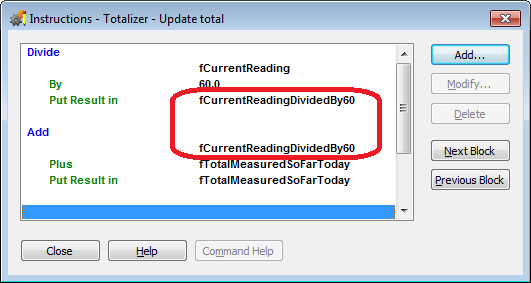# How to Create a Custom Totalizer

Hello,
I have a SNAP-PAC R1 controller and I am pulling in several tables of data via modbus from other devices. I then store the data from these tables into variables.

Since the built in “totalizer” commands seem to only apply to analog input points does anyone know of a way to use Opto Script to write a custom totalizer using a variable as the input?

Thanks!

Ben

Hi Ben,

I’ve been trying to find a sneaky way to use the built-in brain totalizer functions for something that’s not an analog input in your strategy, but no luck.

Can you give us an example of the kind of totalizing you want to do here (size of values, units, how frequently you want to update, etc.)?

The OptoScript I’d be inclined to write would have a loop which perhaps grabs your table element every second, divides by the appropriate amount (like 60 if you’re looking for X units per minute, say), then adds it to your running total. Is that the kind of example you seek?

Thanks,
OptoMary

p.s. Welcome to the OptoForums!

OptoMary,
One example of the type of data I am trying to totalize is a flow rate (gpm, 0-25 gpm). I would like to update this as frequently as possible but have been uncertain of how to ensure my sampling frequency occurs at an exact interval (for example every second or alternatively every 10 seconds). Am I correct in assuming that if I have a countdown timer for every second that the actual interval would could be closer to 1.5 seconds?

I suppose one way around this would be to have a longer sampling interval (10 seconds or 60 seconds) so that a 0.5second error doesn’t make much of a difference.

Also, the variable needs to be reset daily based on the realtime clock.

Thanks,

Ben

Hi Ben,

I’m not sure where you’re getting that 0.5 second error assumption. But then, I wouldn’t use a timer for this either. Here’s how I would do it (just one option, hoping others will share their ideas):

(OptoScript):

``````while (1 == 1) // loop forever

// Get Day & Seconds
nSecondsSinceMidnight = GetSecondsSinceMidnight();
nDay = GetDay();

// See if the day changed since last we looked
if (nDay <> nLastDay) then

// Clear total
fTotalMeasuredSoFarToday = 0;

endif

// See if the seconds changed since we last looked
if (nSecondsSinceMidnight <> nLastSeconds) then

// Update total
fTotalMeasuredSoFarToday = fTotalMeasuredSoFarToday + (fCurrentReading / 60.0);

endif

// Update last day, seconds
nLastSeconds = nSecondsSinceMidnight;
nLastDay = nDay;

// Give other task a chance to run
DelayMsec(1);
wend

``````

If you’re not sure why I have that delay of 1 millisecond in there, check out form 1776 (essentially you’re making sure this chart/task doesn’t hog the CPU).

Here’s how it would look in non-OptoScript blocks:One side note since I’m showing OptoScript vs. Action/Condition blocks here: Notice in the “Update total” Action block, it takes and extra step (and extra variable: fCurrentReadingDividedBy60):vs. the OptoScript equivalent:

fTotalMeasuredSoFarToday = fTotalMeasuredSoFarToday + (fCurrentReading / 60.0);

In spite of my OptoScript bias (as a recovering programmer), I must admit the nice thing about Action/Condition blocks as a flowchart: it’s easy to see at a glance what the logic/flow does. (Maybe even without glasses.)

Here’s this chart in case you’d like to import it in to your PAC Control 9.3 basic or better strategy:
TotalizerChart9.3Basic.zip (1.92 KB)

Hope that helps!
-OptoMary

If the totalizer is embedded in a larger chart, especially if it involves Modbus communication, the loop rate of that chart might be slower. Let’s say it is 50 ms on average, and assume there is at least a few ms random variation in the loop time. Over long time periods, this conditional:

``````  if (nSecondsSinceMidnight <> nLastSeconds) then

// Update total
fTotalMeasuredSoFarToday = fTotalMeasuredSoFarToday + (fCurrentReading / 60.0);

endif
``````

would be satisfied on average every 1.025 sec, introducing some inflation (2.5%) into the calculated total. If you have very predictable loop times you could compensate for this, but an alternative might be to use a timer instead.

In the powerup chart, or on just the first time through the loop, initialize a down timer.

``````SetDownTimerPreset(fTotalizer_Timeout, dtTotalizer_Timer);
StartTimer(dtTotalizer_Timer);
``````

Then, somewhere in the loop that needs the totalizer, include the following:

``````if (dtTotalizer_Timer <> 0) then
fTotalizer = fTotalizer + (fRateInXPerMin * (fTotalizer_Timeout - GetRestartTimer(dtTotalizer_Timer)) / 60.0);
//The timer is reset immediately to keep the elapsed time as accurate as possible.
else
// Deal with a totalizer timeout error
endif

``````

The timeout value should be sufficiently large to cover any possible loop time, but by using a down timer, you are protected against an overflow error if something happens that would cause an up timer to run until it maxes out, as mentioned here: